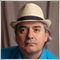# APR, APY and annualized return113

Hello, I am slightly confused regarding the APR, APY and the annualized return based on an exact number of days.

Assuming a starting balance of 10.000 USD after 1500 days (approx. 4.1 years) the balance increased to 35.000 USD. This is an increase of 250%. I calculate the exact (based on 1500 days) annualized return as follows:

(1+250%)^(365/1500)-1=36%

Is it correct that the APR would be the 250% and the APY would be the 36% ?10079APR vs. APY: What’s the Difference?
• www.investopedia.com
It's easy to understand why people may confuse the terms APR and APY. Both are used to calculate interest for investment and credit products. And they significantly affect how much you earn or must pay when they're applied to your account balances. Key Takeaways APR represents the annual rate charged for earning or borrowing money. APY takes...113

Hi Fernando, thank's for your comment and the provided website. I have read that one already but couple of minutes ago I found this website:

https://finance.zacks.com/convert-simple-returns-equity-annualized-returns-1563.html

It is basically a step by step explanation of my formula provided above but in years and not based on exact days but you only have to replace the part at which you divide 1 by the number of years with 365/Number of days and you get your 36%.

HOWEVER, I think this calculated annualized return based on a total percentage increase is different from the APY as for the APY you expect to receive interest on a daily, weekly, monthly or yearly basis which you don't have when you just invest in a stock and hold it for a couple of months or years.

How to Convert Simple Returns on Equity to Annualized Returns
• finance.zacks.com
The simple return on equity refers to the total income divided by the total shareholders' equity over any specified period of time. For example, you use the simple return on equity to find the overall growth rate during the time you held a stock, but because of compounding, you can't simply divide the total return on equity by the number of...